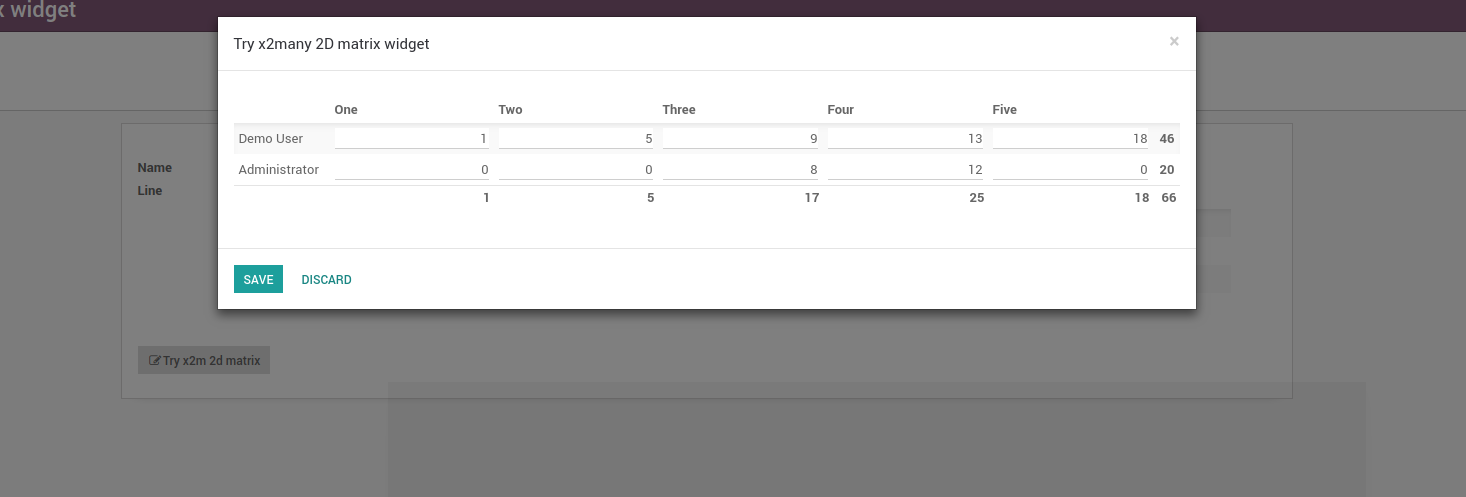# 2D matrix for x2many fields x2many字段的2D矩阵

## x2many字段的2D矩阵

### 此模块允许在表中显示带有3元组（\$ x_value，\$ y_value，\$ value）的x2many字段

This module allows to show an x2many field with 3-tuples (\$x_value, \$y_value, \$value) in a table

\$x_value1\$x_value2
\$y_value1\$value(1/1)\$value(2/1)
\$y_value2\$value(1/2)\$value(2/2)

where value(n/n) is editable.

An example use case would be: Select some projects and some employees so that a manager can easily fill in the planned_hours for one task per employee. The result could look like this:The beauty of this is that you have an arbitrary amount of columns with this widget, trying to get this in standard x2many lists involves some quite ugly hacks.

## Usage

Use this widget by saying:

```<field name="my_field" widget="x2many_2d_matrix" />
```

This assumes that my_field refers to a model with the fields xy and value. If your fields are named differently, pass the correct names as attributes:

```<field name="my_field" widget="x2many_2d_matrix" field_x_axis="my_field1" field_y_axis="my_field2" field_value="my_field3">
<tree>
<field name="my_field"/>
<field name="my_field1"/>
<field name="my_field2"/>
<field name="my_field3"/>
</tree>
</field>
```

You can pass the following parameters:

field_x_axis
The field that indicates the x value of a point
field_y_axis
The field that indicates the y value of a point
field_label_x_axis
Use another field to display in the table header
field_label_y_axis
Use another field to display in the table header
field_value
Show this field as value
show_row_totals
If field_value is a numeric field, it indicates if you want to calculate row totals. True by default
show_column_totals
If field_value is a numeric field, it indicates if you want to calculate column totals. True by default

### Example

You need a data structure already filled with values. Let's assume we want to use this widget in a wizard that lets the user fill in planned hours for one task per project per user. In this case, we can use project.task as our data model and point to it from our wizard. The crucial part is that we fill the field in the default function:

```from odoo import fields, models

class MyWizard(models.TransientModel):
_name = 'my.wizard'

# your list of project should come from the context, some selection
# in a previous wizard or wherever else
projects = self.env['project.project'].browse([1, 2, 3])
# same with users
users = self.env['res.users'].browse([1, 2, 3])
return [
(0, 0, {
'project_id': p.id,
'user_id': u.id,
'planned_hours': 0,
'message_needaction': False,
})
# if the project doesn't have a task for the user,
# create a new one
if not p.task_ids.filtered(lambda x: x.user_id == u) else
(4, p.task_ids.filtered(lambda x: x.user_id == u).id)
for p in projects
for u in users
]

```<field name="task_ids" widget="x2many_2d_matrix" field_x_axis="project_id" field_y_axis="user_id" field_value="planned_hours">